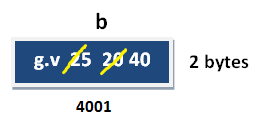# Variable in C

## Variable in C Language

Variable is an identifier which holds data or another one variable. It is an identifier whose value can be changed at the execution time of program. It is used to identify input data in a program.

Syntax: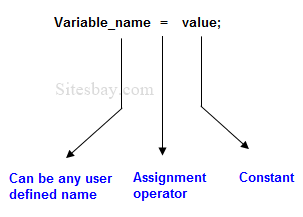## Syntax

```Variable_name = value;
```

### Rules to declare a Variable

To Declare any variable in C language you need to follow rules and regulation of C Language, which is given below;

• No spaces are allowed in variable declaration.
• Except underscore (_) no other special symbol are allowed in the middle of the variable declaration (not allowed -> roll-no, allowed -> roll_no).
• Maximum length of variable is 8 characters depend on compiler and operation system.
• Every variable name always should exist in the left hand side of assignment operator (invalid -> 10=a; valid -> a=10;).
• No keyword should access variable name (int for <- invalid because for is keyword).

Note: In a c program variable name always can be used to identify the input or output data.

## Variable declarations

This is the process of allocating sufficient memory space for the data in term of variable.

## Syntax

Datatype variable_name; int a;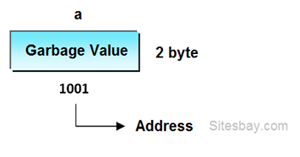If no input values are assigned by the user than system will gives a default value called garbage value.

## Garbage value

Garbage value can be any value given by system and that is no way related to correct programs. This is a disadvantage of C programming language and in C programming it can overcome using variable initialization.

## Variable initialization

It is the process of allocating sufficient memory space with user defined values.

## Syntax

```Datatype nariable_name=value;
```

## Example

```int  b = 30;
```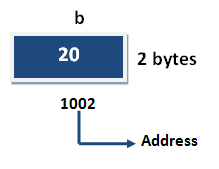## Variable assignment

It is a process of assigning a value to a variable.

## Syntax

Variable_Name = value

## Example

```int  a= 20;
int b;
```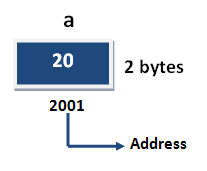## Example

```b = 25; // --> direct assigned variable
b = a;  // --> assigned value in term of variable
b = a+15;  // --> assigned value as term of expression
```